Mean Energy Loss/Average Energy Loss Per Inelastic Electron Collision
- Practical Electron Microscopy and Database -
- An Online Book -
Microanalysis | EM Book                                                                                   http://www.globalsino.com/EM/

=================================================================================

The mean energy transfer (loss) per inelastic collision of a charged particle upon penetration through matter is given by the ratio of the stopping power to σtot, which is approximately given by the ratio of the leading coefficients. Here, σtot is the total inelastic-scattering cross section. The mean energy transfer per unit path length is presented by the stopping power given by the well-known Bethe formula. [1-3]

Based on Poisson’s Law, the average energy loss,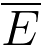, per incident electron can be given by,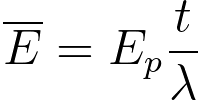----------------------------------------- [960a]
where,
λ -- the mean free path of electrons at a specific accelerating voltage,
t -- the local TEM sample thickness,
Ep – the plasmon energy.

The average energy loss for a core-loss can be given by,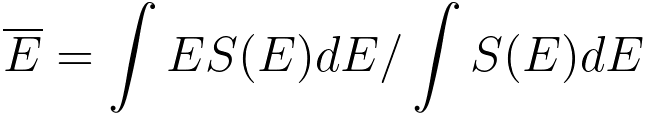--------------------- [960b]
where,
S(E) -- the signal intensity at an energy loss E.

Assuming the core-loss intensity is proportional to E-s, then, Egerton theoretically simplified Equation 960b to the following equation,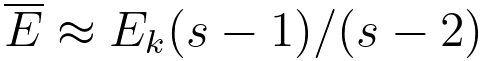----------------------------------------- [960c]
where,
Ek -- the energy of core-loss,
s - a constant between 2 and 4.

Figure 960a shows the mean energy, Eav, transferred to an atom per inelastic collision of a fast charged particle with atoms plotted against the atomic number Z. Table 960a lists some mean energy losses per inelastic electron collision with some numbers extracted from Figure 960a. The mean energy transfer per any collision is roughly ~68 eV on the average over all atoms with Z ≤ 38.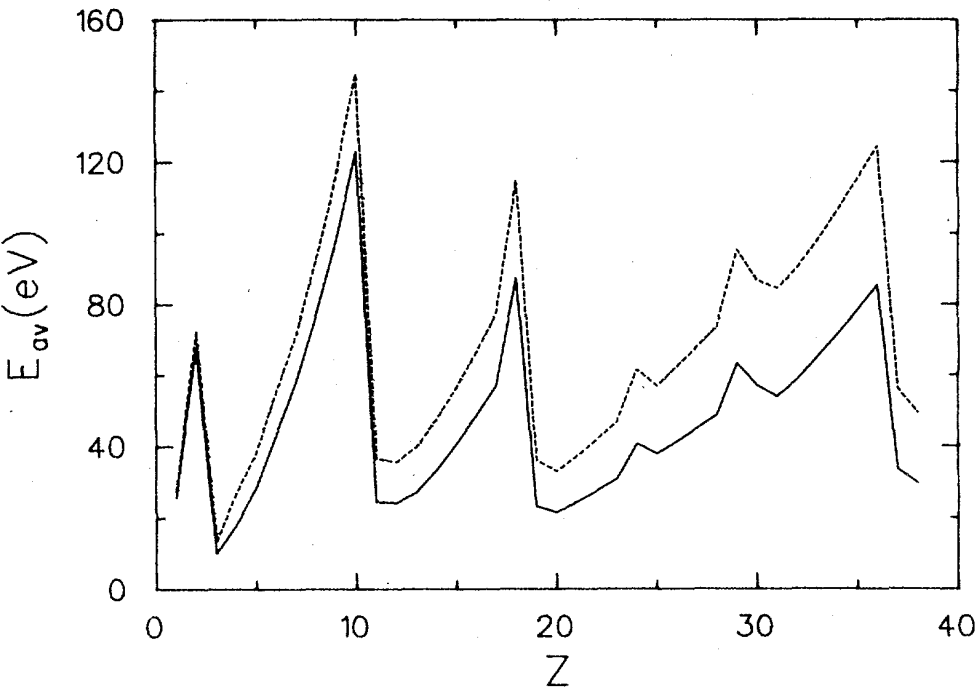Figure 960a. Mean energy, Eav, transferred to an atom per inelastic collision of a fast charged particle with atoms plotted against the atomic number Z.  The broken curve shows the plots at extremely high energies, while the solid line shows the curve at β= 0.4127 (which corresponds to 50-keV electrons or to 918.1-MeV protons). Here, β = c/v, v is the relativistic speed of the charged particle, and c is the speed of light.

 Table 960a. Mean energy, Eav, transferred to an atom per inelastic collision of a fast charged particle with atoms and materials against the atomic number Z.
Atomic number, Z
Element
Mean energy loss (eV)
Atomic number, Z
Element
Mean energy loss (eV)
Atomic number, Z
Materials
Mean energy loss (eV)
5 B 30 19 K 22 49 ZrO2
6 C 48 22 Ti 27
7 N 68 28 Ni 50
8 O 88 29 Cu 62
13 Al 29 32 Ge 60
14 Si 35 74 W

 Table 960b. Mean energy, Eav, transferred to an atom per inelastic collision of a fast charged particle with materials against the atomic number Z.
Materials
Charged particle
Energy of the charged particle Mean energy loss (eV)   Materials
Charged particle
Energy of the charged particle
Mean energy loss (eV)
ZrO2   Proton 0.5 MeV 61.4
1 MeV 71.7
5 MeV 94.1
10 MeV 101.9
100 MeV 117.1

 H. Bethe, Ann. Phys. (Leipzig) 5, 325 (1930).
 H. Bethe, in IIandblch de~ Physik, edited by H. Geiger and K. Scheel (Springer, Berlin, 1933), Vol. 24/1, p 273.
 U. Fano, Ann. Rev. Nucl. Sci. 13, 1 (1963).
 Mitio Inokuti, J. L. Dehmer, T. Sacr, and J.D. Hanson, Oscillator-strength moments, stopping powers, and total inelastic-scattering cross sections of all atoms through strontium, Physical Reveiw A, 23 (1), (1981).
 Jennifer Schofield, Simon M. Pimblott, Inelastic cross-sections and energy loss properties by non-relativistic heavy ions in zirconium dioxide, 372 (1), 2016, p119-126.
 Egerton R.F., Electron Energy Loss Spectroscopy in the Electron Microscope (NewYork, Plenum Press, 1986).

=================================================================================